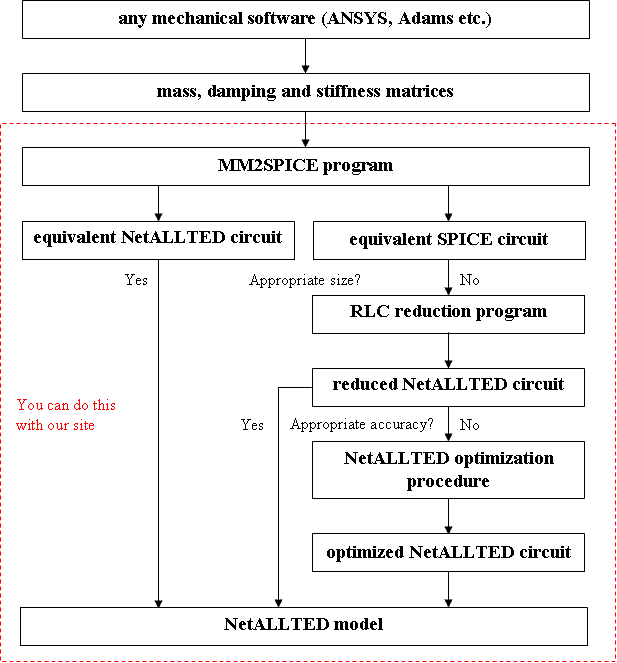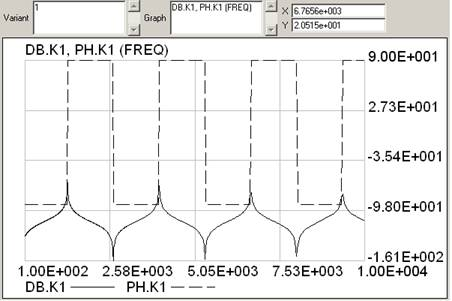DownloadDocumentationModelling

## What you can do with this site?

There are some ways how to get a NetALLTED model from a finite-element model prepared by means of any mechanical software from which it is possible to get mass, damping and stiffness matrices describing the system status in MarketMatrix format (Fig. 1).Fig. 1. The process to get a NetALLTED model

The process to build a mechanical object.s model can go by three different ways subject to dimension and accuracy required.

Way 1. You can get a model equivalent by the accuracy to the source mechanical object described in mechanical software. In the most cases, it is used only to check that the simulation results in NetALLTED coincide with those obtained by mechanical software since the real usage of such model is difficult due to its large dimension. So, after completing Way 1, in the most cases you should proceed to Way 2.

Way 2. Here, you can make use of the RLC reduction methods in order to get a reduced circuit whose accuracy and dimension depend on the MAX_TAU value. If you do not satisfy with the accuracy of such model (by the dimension required) you should proceed to Way 3.

Way 3. Here, you can make use of the NetALLTED optimization procedures in order to adjust the accuracy of a model to the desired value.

The step-by-step instructions how to make use of the above-described ways at this site are presented below.

## The process to get an equivalent circuit

1. Copy mass and stifness (K and M) matrices into the files at a disk (for example, test.K, test.M).
2. Go to the MM2SPICE tab.
3. At the input fields, indicate the path to the respective files and press the Execute button.
4. Copy the obtained circuit description files onto a disk (for example, test.cir, test.atd).
5. Simulation of the equivalent circuit:

6. 5.1) Add the following lines to the beginning of the description file test.atd:
 Task Option 47, 48; && Object Circuit 1d; J1(100,0)=-100;
5.2) Add the following lines to the end of the description file test.atd:
 && task dc; ac; tf K1=UJ1/IJ1; lplot db.K1, ph.K1(1000); const lfreq=100, ufreq=10000; && end
5.3) Go to the NetALLTED tab.
5.4) Indicate the path to the task file (test.atd), or copy text into the input field and press the Execute button.

If all is right, you have to see the following picture:Using a cursor to get values of the resonance frequencies, you can make sure that the obtained equivalent circuit has the following characteristics in a work range (see Table 1).

## The process to get a reduced circuit

1. Go to the RLC tab.
2. Copy the following text into the file (for example, test.rc):
3.  MAX_TAU 3e-5 KEEP 0 KEEP 100
4. At the input fields, indicate the path to the respective files (test.cir, test.rc) and press the Execute button.
5. Copy the obtained circuit into the file (for example, test_reduce.atd).
6. Simulation of the reduced equivalent circuit:

7. 5.1) Add the following lines to the beginning of the description file test_reduce.atd:
 Task Option 47, 48; && Object Circuit 1d; J1(100,0)=-100;
5.2) Add the following lines to the end of the description file test_reduce.atd:
 && task dc; ac; tf K1=UJ1/IJ1; lplot db.K1, ph.K1(1000); const lfreq=100, ufreq=10000; && end
5.3) Go to the NetALLTED tab.
5.4) Indicate the path to the task file (test_reduce.atd), or copy text into the input field and press the Execute button.

If all is right, you have to see the following picture:Using a cursor to get values of the resonance frequencies, you can make sure that the obtained reduced circuit has the following characteristics in a work range (see Table 1).

## The process to get an optimized circuit

If the obtained accuracy of a reduced model is not enough, it is possible to use one of two approaches:

1. Obtaining an equivalent reduced circuit at the lower values of. Thus, the circuit got under= 5e-6 has a lower error (see Table 1). However, in this case, the size of an equivalent reduced circuit increases.
2. Optimization of a circuit by means of the respective possibilities of the circuit design software.

Let.s assume that we know the required values of the construction.s eigenfrequencies (for example, after simulation by ANSYS).
Using test_reduce.atd as a source circuit, let.s formulate a task in the following way: to get the summary error at the two first peaks less than 0.1%. In this case, a task file for optimization looks like:

 Object Circuit Beam; J1(100,0)=-100; C_1(82,100) = -0.116667; L_10(100,0) = -34161.8; L_11(23,50) = 1.35e-10; L_12(23,0) = 1.15e-10; L_13(50,0) = -16435.9; L_14(50,82) = 1.6e-10; C_2(100,0) = 6.3; C_3(23,50) = -0.116667; C_4(23,0) = 17.3833; C_5(50,82) = -0.116667; C_6(0,50) = 20.65; C_7(0,82) = 17.5; L_8(82,100) = 9e-11; L_9(82,0) = -580750; && task dc; ac; OPTIM; OPTION 47,48; tf K1=UJ1/IJ1; FIX T1=MAXA(db.K1,100,1600), T2=MAXA(db.K1,1700,4100); OF ERROR1=F8(1336.3,4009.3/T1,T2); VARPAR L_8(8e-11,1e-10); VARPAR L_11(1e-10,2e-10); VARPAR L_12(1e-10,2e-10); VARPAR L_14(1e-10,2e-10); lplot db.K1,ph.K1(1200); const lfreq=100, ufreq=4100; CONST METHOD=40, numb=100; const operr=1e-6; && end

Go to the NetALLTED tab, copy this text into the input field and press the Execute button.

As you can see from optimization results, it is possible to ajust the macromodel in a narrow frequency range to the desired accuracy; the summary error is equal to 0.07% in this case (see Table 1).

The advantage of such approach is a small size of the equivalent reduced circuit as well as, under a narrow range of working frequencies, the possibility to get the required frequencies with a high accuracy. The disadvantage is a necessity to carry out an additional analysis of the circuit in order to select the most sensitive elements. When the reduced circuit has too small size, the sensitivity of each element increases resulting in the complexity both to define variable parameters. changing range and to find global optimum by optimization methods. So, using this approach, it is recommended to use random search methods (method=40) with a large sampling dimension.

Table 1.

 Equivalent circuit Reduced circuit Optimized circuit- 10-5 3*10-5 3*10-5 Number of nodes 101 12 5 5 Number of elements 314 38 14 14 Reduction by nodes, % - 88.1188 95.0495 95.0495 Reduction by elements, % - 87.8981 95.5414 95.5414 1st peak, Hz 1336.3 1334.5 1328.0 1336.2 2nd peak, Hz 4009.3 3982.7 3662.2 4012.2 3rd peak, Hz 6683.2 6608.4 5607.9 - 4th peak, Hz 9358.8 9345.3 8110.8 -

Attention! At the current moment, the NetALLTED simulation graphical results are outputted in a single scale and could not match with those presented in the documentation.